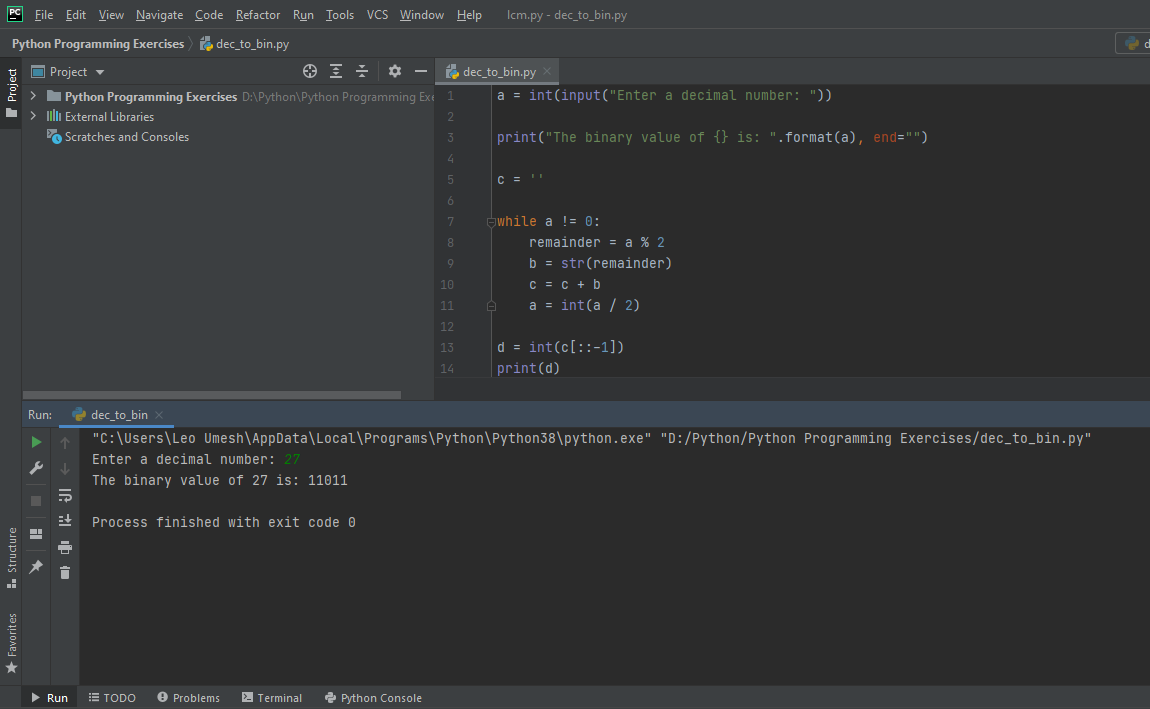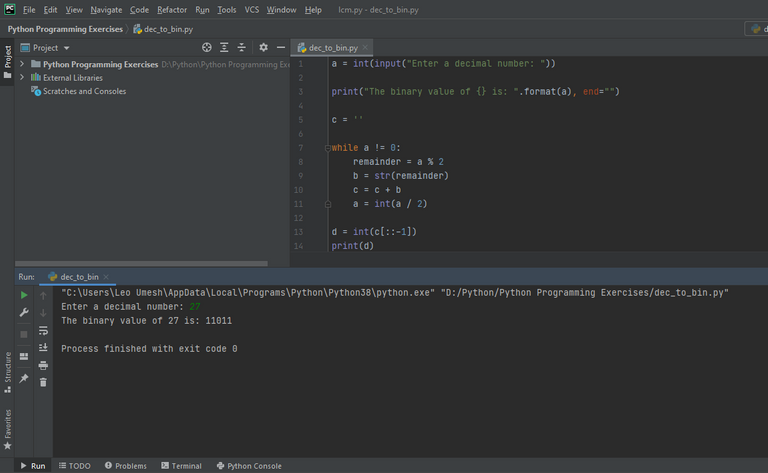# Converting Decimal Number To Binary Number In Python

in STEMGeeks2 years ago

In mathematics and computer science, binary number are those number having the base of 2. In other words, they are written interms of 0 and 1 or machine code that only computer can understand in order to execute certain tasks. The following example is a code in python that asks user to enter the number in order to find its binary equivalent and also prints the result. The logic is very simple inorder to calculate the same. In python, you have in-built function to perform certain task within a second but I always prefer learning through my own way as it helps to sharpen your problem solving skills and also increases your understanding of various concepts.

``````a = int(input("Enter a decimal number: "))

print("The binary value of {} is: ".format(a), end="")

c = ''

while a != 0:
remainder = a % 2
b = str(remainder)
c = c + b
a = int(a / 2)

d = int(c[::-1])
print(d)
``````

We asked for input from user and then used a while loop to find the remainder. We created an empty string c at the beginning of the program. Inside while loop unless the condition becomes false, the empty string concatenates with the remainder. And we need to run the loop until the dividend becomes 0. In python, the division is pretty straightforward. You can divide any number without specifying whether it is integer value, float value, double value and so on. So I used int() function to convert the dividend to integer. After coming out of while loop, we reverse the string in order to get our desired output using the concept of slice.

Lets try executing this code by converting 27 into its equivlent binary number which is 11011. You can see the same output:Converting Decimal Number To Binary Number In Python

In mathematics and computer science, binary number are those number having the base of 2. In other words, they are written interms of 0 and 1 or machine code that only computer can understand in order to execute certain tasks. The following example is a code in python that asks user to enter the number in order to find its binary equivalent and also prints the result. The logic is very simple inorder to calculate the same. In python, you have in-built function to perform certain task within a second but I always prefer learning through my own way as it helps to sharpen your problem solving skills and also increases your understanding of various concepts.

``````a = int(input("Enter a decimal number: "))

print("The binary value of {} is: ".format(a), end="")

c = ''

while a != 0:
remainder = a % 2
b = str(remainder)
c = c + b
a = int(a / 2)

d = int(c[::-1])
print(d)
``````

We asked for input from user and then used a while loop to find the remainder. We created an empty string c at the beginning of the program. Inside while loop unless the condition becomes false, the empty string concatenates with the remainder. And we need to run the loop until the dividend becomes 0. In python, the division is pretty straightforward. You can divide any number without specifying whether it is integer value, float value, double value and so on. So I used int() function to convert the dividend to integer. After coming out of while loop, we reverse the string in order to get our desired output using the concept of slice.

Lets try executing this code by converting 27 into its equivlent binary number which is 11011. You can see the same output:Sort:
2 years ago Reveal Comment